Home Practice
For learners and parents For teachers and schools
Textbooks
Full catalogue
Pricing SupportLog in

We think you are located in United States. Is this correct?

# 8.2 Ratio and proportion

## 8.2 Ratio and proportion (EMCJ8)

temp text

Ratio

A ratio describes the relationship between two quantities which have the same units. We can use ratios to compare weights, heights, lengths, currencies, etc. A ratio is a comparison between two quantities of the same kind and has no units.

Example: if the length of a rectangle is $$\text{20}$$ $$\text{cm}$$ and the width is $$\text{60}$$ $$\text{cm}$$, then we can express the ratio between the length and width of the rectangle as:

\begin{align*} \text{length to width } &= 20 \text{ to } 60 \\ &= 1 \text{ to } 3 \\ & \\ \text {Or } \quad \frac{\text{length}}{\text{width}} &= \frac{20}{60} \\ &= \frac{1}{3} \\ & \\ \text {Or } \quad \text{length} : \text{width } &= 20: 60 \\ &= 1: 3 \end{align*}
• The ratio of $$\frac{1}{3}$$ describes the length of the rectangle relative to its width.
• A ratio written as a fraction is usually given in its simplest form.
• A ratio gives no indication of actual length. For example,

$\frac{\text{length}}{\text{width}} = \frac{\text{50}\text{ cm}}{\text{150}\text{ cm}} \enspace \text{also gives a ratio of} \enspace \frac{1}{3}$ $\text{And} \enspace \frac{\text{length}}{\text{width}} = \frac{\text{0,8}\text{ m}}{\text{2,4}\text{ m}} \enspace \text{also gives a ratio of} \enspace \frac{1}{3}$
• Do not convert a ratio to a decimal (even though $$\frac{1}{3}$$ and $$\text{0,}\dot{3}$$ have the same numerical value).

Proportion

## Predicting heights

A record of heights is given.

If the ratio $$\frac{\text{height of a person at two years old}} {\text{height of a person as an adult}}$$ is $$\text{1}$$ to $$\text{2}$$, complete the table below:

 Name Height at two years Height as an adult Hendrik $$\text{84}$$ $$\text{cm}$$ Kagiso $$\text{162}$$ $$\text{cm}$$ Linda $$\text{86}$$ $$\text{cm}$$ Mandisa $$\text{0,87}$$ $$\text{m}$$ Prashna $$\text{1}$$ $$\text{m}$$ $$\text{64}$$ $$\text{cm}$$

Consider the diagram below: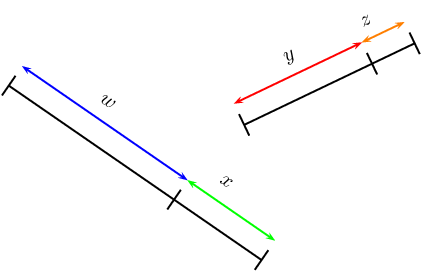If two or more ratios are equal to each other, then we say that they are in the same proportion. Proportionality describes the equality of ratios.

If $$\dfrac{w}{x} = \dfrac{y}{z}$$, then $$w$$ and $$x$$ are in the same proportion as $$y$$ and $$z$$.

1. $$wz = xy$$
2. $$\dfrac{x}{w} = \dfrac{z}{y}$$
3. $$\dfrac{w}{y} = \dfrac{x}{z}$$
4. $$\dfrac{y}{w} = \dfrac{z}{x}$$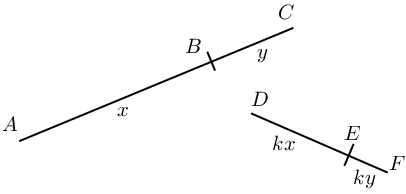Given

$\frac{AB}{BC}=\frac{x}{y}=\frac{kx}{ky}=\frac{DE}{EF}$

The line segments $$AB$$ and $$BC$$ are in the same proportion as $$DE$$ and $$EF$$. The following statements are also true:

 Proportion Reciprocal proportion Cross multiplication $$\frac{AB}{BC}=\frac{DE}{FE}$$ $$\frac{BC}{AB}=\frac{FE}{DE}$$ $$AB \cdot FE = BC \cdot DE$$ $$\frac{AB}{AC}=\frac{DE}{DF}$$ $$\frac{AC}{AB}=\frac{DF}{DE}$$ $$AB \cdot DF = AC \cdot DE$$ $$\frac{BC}{AC}=\frac{EF}{DF}$$ $$\frac{AC}{BC}=\frac{DF}{EF}$$ $$BC \cdot DF = AC \cdot EF$$

We can also substitute $$x$$, $$y$$, $$kx$$, and $$ky$$ to show algebraically that the statements are true.

For example,

\begin{align*} BC \cdot DF &= y \times (kx + ky) \\ &= ky (x + y) \\ \text{And } AC \cdot EF &= (x+y) \times ky \\ &= ky(x+y) \\ \therefore BC \cdot DF &= AC \cdot EF \end{align*}

## Ratio and proportion

Textbook Exercise 8.2

Solve for $$p$$:

$$\frac{8}{40} = \frac{p}{25}$$
\begin{align*} \frac{8}{40} &= \frac{p}{25} \\ \frac{8 \times 25}{40} &= p \\ \frac{200}{40} &= p \\ \therefore 5 &= p \end{align*}
$$\frac{6}{9} = \frac{29 + p}{54}$$
\begin{align*} \frac{6}{9} &= \frac{29 + p}{54} \\ \frac{6 \times 54}{9} &= 29 + p \\ 36 &= 29 + p \\ \therefore 7 &= p \end{align*}
$$\frac{3}{1 + \frac{p}{4}} = \frac{4}{p + 1}$$
\begin{align*} \frac{3}{1 + \frac{p}{4}} &= \frac{4}{p + 1} \\ 3(p + 1) &= 4 \left( 1 + \frac{p}{4} \right) \\ 3p + 3 &= 4 + p \\ 2p &= 1 \\ \therefore p &= \frac{1}{2} \end{align*}
$$\frac{14}{100 - p} = \frac{49}{343}$$
\begin{align*} \frac{14}{100 - p} &= \frac{49}{343} \\ 14 \times 343 &= 49( 100 - p) \\ \frac{4802}{49} &= 100 - p \\ 98 &= 100 - p \\ \therefore p &= 2 \end{align*}

A packet of $$\text{160}$$ sweets contains red, blue and yellow sweets in the ratio of $$3:2:3$$ respectively. Determine how many sweets of each colour there are in the packet.

\begin{align*} 3 + 2 + 3 &= 8 \\ \text{Red } &= \frac{3}{8} \times 160 \\ &= 60 \\ \text{Blue } &= \frac{2}{8} \times 160 \\ &= 40 \\ \text{Yellow } &= \frac{3}{8} \times 160 \\ &= 60 \end{align*}

A mixture contains $$\text{2}$$ parts of substance $$A$$ for every $$\text{5}$$ parts of substance $$B$$. If the total weight of the mixture is $$\text{50}$$ $$\text{kg}$$, determine how much of substance $$B$$ is in the mixture (correct to $$\text{2}$$ decimal places).

\begin{align*} \text{Ratio substance } A \text{ to substance B } &= 2 : 5 \\ 2 + 5 &= 7 \\ \text{Substance } B &= \frac{5}{7} \times 50 \\ &= \text{35,71}\text{ kg} \end{align*}

Given the diagram below.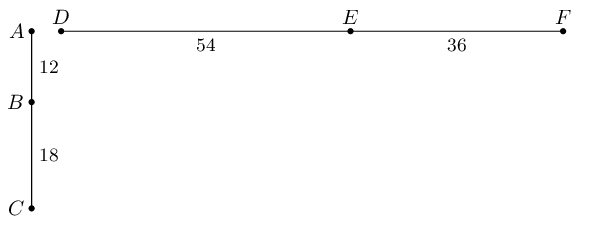Show that:

$$\frac{AB}{BC} = \frac{FE}{ED}$$
\begin{align*} \frac{AB}{BC} &= \frac{12}{18} \\ &= \frac{2}{3} \\ \frac{FE}{ED} &= \frac{36}{54} \\ &= \frac{2}{3} \\ \therefore \frac{AB}{BC} &= \frac{FE}{ED} \end{align*}
$$\frac{AC}{BC} = \frac{FD}{EF}$$
\begin{align*} \frac{AC}{BC} &= \frac{12 + 18}{18} \\ &= \frac{30}{18} \\ &= \frac{5}{3} \\ \frac{FD}{EF} &= \frac{36 + 54}{54} \\ &= \frac{90}{54} \\ &= \frac{5}{3} \\ \therefore \frac{AC}{BC} &= \frac{FD}{EF} \end{align*}
$$AB \cdot DF = AC \cdot FE$$
\begin{align*} AB \cdot DF &= 12 \times (36 + 54) \\ &= 12 \times 90 \\ &= 1080 \\ AC \cdot FE &= (12 + 18) \times 36 \\ &= 30 \times 36 \\ &= 1080 \\ \therefore AB \cdot DF &= AC \cdot FE \end{align*}

Consider the line segment shown below.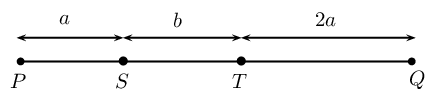Express the following in terms of $$a$$ and $$b$$:

$$PT: ST$$
$$(a + b): b$$
$$\frac{PS}{TQ}$$
$$\frac{a}{2a} = \frac{1}{2}$$
$$\frac{SQ}{PQ}$$
$$\frac{2a + b}{3a + b}$$
$$QT: TS$$
$$2a: b$$

$$ABCD$$ is a parallelogram with $$DC = \text{15}\text{ cm}$$, $$h = \text{8}\text{ cm}$$ and $$BF = \text{9}\text{ cm}$$.

Calculate the ratio $$\dfrac{\text{area } ABF}{\text{area } ABCD}$$.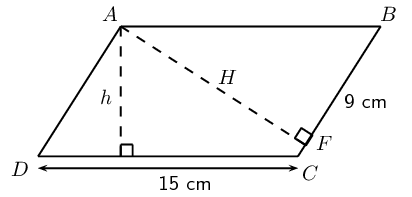The area of a parallelogram $$ABCD =$$ base $$\times$$ height:

\begin{align*} \text{Area} &= \text{15} \times \text{8} \\ &= \text{120}\text{ cm$^{2}$} \end{align*}

The perimeter of a parallelogram $$ABCD = 2DC + 2BC$$.

To find the length of $$AF$$, we use $$AF \perp BC$$ and the theorem of Pythagoras.

\begin{align*} \text{In $$\triangle ABF$$:} \quad AF^2 &= AB^2 - BF^2 \\ &= \text{15}^2 - \text{9}^2 \\ &= \text{144} \\ \therefore AF &= \text{12}\text{ cm} \\ \therefore \text{area } ABF &= \frac{1}{2} AF \cdot BF \\ &= \frac{1}{2}(12)(9)\\ &= \text{54}\text{ cm} \end{align*} \begin{align*} \therefore \frac{\text{area } ABF}{\text{area } ABCD} &= \frac{54}{120} \\ &= \frac{9}{20} \end{align*}

$$AB = \text{36}\text{ m}$$ and $$C$$ divides $$AB$$ in the ratio $$4:5$$. Determine $$AC$$ and $$CB$$.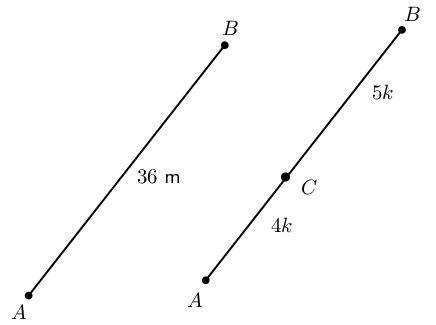\begin{align*} \frac{AC}{AB} &= \frac{4k}{(4k + 5k)} \\ \therefore AC &= 36 \times \frac{4}{9} \\ &= \text{16}\text{ m} \\ CB &= 36 - 16 \\ &= \text{20}\text{ m} \\ \text{Or } \frac{CB}{AC} &= \frac{5k}{(4k + 5k)} \\ \therefore CB &= 36 \times \frac{5}{9} \\ &= \text{20}\text{ m} \end{align*}

If $$PQ = \text{45}\text{ mm}$$ and the ratio of $$TQ:PQ$$ is $$2:3$$, calculate $$PT$$ and $$TQ$$.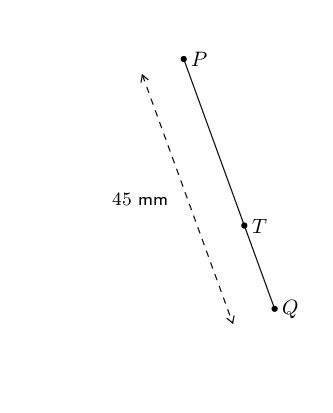\begin{align*} \frac{TQ}{PQ} &= \frac{2}{3} \\ \therefore TQ &= PQ \times \frac{2}{3} \\ &= 45 \times \frac{2}{3} \\ &= \text{30}\text{ mm} \\ PT &= 45 - 30 \\ &= \text{15}\text{ mm} \\ \text{Or } \quad \frac{PT}{PQ} &= \frac{1}{3} \\ \therefore PT &= 45 \times \frac{1}{3} \\ &= \text{15}\text{ mm} \end{align*}

Luke's biology notebook is $$\text{30}$$ $$\text{cm}$$ long and $$\text{20}$$ $$\text{cm}$$ wide. The dimensions of his desk are in the same proportion as the dimensions of his notebook.

If the desk is $$\text{90}\text{ cm}$$ wide, calculate the area of the top of the desk.

\begin{align*} \text{Ratio } &= \frac{\text{table width}}{\text{book width}} \\ &=\frac{90}{20} \\ &=\text{4,5} \\ \therefore \text{Length of table } &= 30 \times \text{4,5} \text{cm} \\ &=\text{135}\text{ cm}\\ \text{Area table} &= 135 \times 90 \\ &=\text{12 150}\text{ cm$^{2}$}\\ &=\text{1,2}\text{ m$^{2}$} \end{align*}

Luke covers each corner of his desk with an isosceles triangle of cardboard, as shown in the diagram: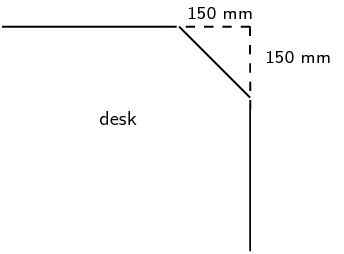Calculate the new perimeter and area of the visible part of the top of his desk.

\begin{align*} x^2&=15^2+15^2 \\ x&=\text{21,2}\text{ cm}\\ \text{New length}&=135-2(15) \\ &=\text{105}\text{ cm} \\ \text{New breadth}&=90-2(15) \\ &=\text{60}\text{ cm}\\ \text{New perimeter }&=2(105)+2(60)+4(\text{21,2})\\ &=\text{414,8}\text{ cm} \end{align*} \begin{align*} \text{Area cut off}&=2\times(15^2) \\ &=\text{450}\text{ m$^{2}$} \\ \text{New area } &= \text{12 150}\text{ cm$^{2}$} - \text{450}\text{ m$^{2}$} \\ &= \text{11 700}\text{ cm$^{2}$} \end{align*}
Use this new area to calculate the dimensions of a square desk with the same desk top area.
\begin{align*} s^2&= \text{11 700}\text{ cm$^{2}$} \\ \therefore s&= \text{108,2}\text{ cm}\\ \text{Square table of length} &\approx 108 \times 108 \text{cm$^{2}$} \end{align*}